# On Optimization of Inventory Management of an Industrial Enterprise. On Analytical Approach for Prognosis of Processes

On Optimization of Inventory Management of an Industrial Enterprise. On Analytical Approach for Prognosis of Processes
Nizhny Novgorod State University, 23 Gagarin avenue, Nizhny Novgorod, 603950, Russia

Nizhny Novgorod State Technical University, 24 Minin Street, Nizhny Novgorod, 603950, Russia

Corresponding Author Email:
elp2004@mail.ru
Page:
26-29
|
DOI:
https://doi.org/10.18280/ama_a.560105
5 January 2019
|
Accepted:
6 March 2019
|
Published:
31 March 2019
| Citation

OPEN ACCESS

Abstract:

In this paper we introduce a model for analysis of inventory management. Based on this model and procedure of analysis of function on extremum we formulate a condition to minimize of attendant costs.

Keywords:

minimization of attendant costs, inventory management, industrial enterprise, prognosis of processes, analytical approach

1. Introduction

The necessity of maximization of profits leads to the need to compile an optimal output plan with available resources and to decrease costs of products. One of the forms of costs is the cost of storing stocks. With their increase, this value also increases. At the same time, a decrease in the supply of resources can lead to a halt in production.

For prognosis of economic processes, it is necessary to have models of this process. At the same time, it is necessary to have approaches for analysis of above models. Some appropriate models have been considered in Refs. [1-5]. In the present paper we introduce a model of enterprise inventory management in more common case in comparison with recently introduced models. Based on this model a compromise value of the parameters was obtained, which makes it possible to minimize the costs associated with the availability of reserves.

2. Pmethod of Solution

We consider the equation of costs in the following form

$I=\frac{a\,b}{x}+b\,c+d\,x$.       (1)

Here x is the quantity of the considered product, a is the organizational cost, b is the intensity of demand, c is the cost of the products, d is the cost of maintaining of products. The first term of the function (1) describes the general organizational costs, the second term of the function (1) describes the cost of the products, the third term of the function (1) describes the total costs of maintaining the stocks. Parameters a, b, c and d are assumed to be constant and known parameters. We determine the quantity of the consignment corresponding to the minimum in the framework of the standard procedure for finding the extremum of the function , i.e. from the condition that the derivative of cost function I by the product quantity x is equal to zero. This condition can be represented in the following form

$\frac{d\,I}{d\,x}=-\frac{a\,b}{{{x}^{2}}}+d=0$.         (2)

Solution of this equation could be written in the following form: $x_0=√(ab⁄d)$. The quantity of product corresponds to the minimum of cost of the enterprise. Dependences of the cost function (1) on the product quantity are shown in Figures 1. Figure 1a shows dependences of the cost function on the product quantity at different values of organizational cost a. Increasing of number of curves numbers corresponds to increasing of value of organizational cost. The figure shows that increasing of organizational cost leads to increasing of the considered cost function. One can find maximal difference of cost function at different value of organizational cost near optimal value of product quantity x0. Increasing and decreasing of organizational cost leads to decreasing of difference between values of cost function with different values of organizational cost. Figure 1b illustrates dependences of the cost function on the product quantity at different values of intensity of demand b. Increasing of number of curves numbers again corresponds to increasing of value of intensity of demand. In this situation one can find again maximal difference of cost function at different value of intensity of demand near optimal value of product quantity x0. Increasing and decreasing of organizational cost leads to decreasing of difference between values of cost function with different values of intensity of demand. However the difference between different values of cost function at different values of intensity of demand is larger than at different values of organizational cost. Figure 1c illustrates dependences of the cost function on the product quantity at different values of cost of the products c. In this situation one can find the similar trends as for previous figures. However in area of small values of product quantity one can find fast rendezvous of all curves. At the same time in area of large values of product quantity one can find almost parallel lines for all dependences. Figure 1d illustrates dependences of the cost function on the product quantity at different values of maintaining of products d. In this situation one can find the similar trends as for Figures 1a and 1b again. In area of small values of product quantity one can find fast rendezvous of all curves anew. However Figures. 1d shows essential expansion of increasing of cost function with increasing of maintaining of products.

## 1a.pngFigure 1a. Dependence of the cost function on the quantity of product at different values of organizational cost a

## 1b.png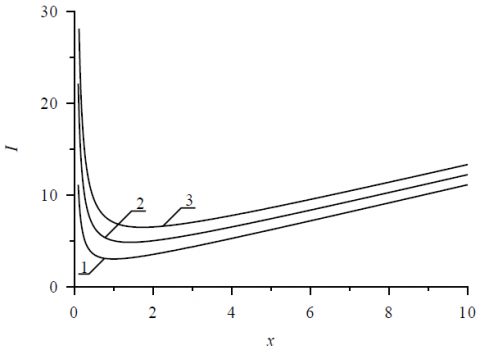Figure 1b. Dependence of the cost function on the quantity of product at different values of intensity of demand b

## 1c.pngFigure 1c. Dependence of the cost function on the quantity of product at different values of cost of the products c

As quantitative characteristics of changing of the cost function (1) it could be used derivative of the function on the product quantity (2). Figures 2 illustrates the derivative. Figure 2a illustrates dependences of the considered derivative of the cost function on the product quantity at different values of organizational cost a. Increasing of number of curves numbers corresponds to increasing of value of organizational cost. The figure shows increasing of the cost function with increasing of organizational cost. The dependence is enough clear framework economic theory. Increasing of quantity of product x before minimum of cost leads to decreasing of cost function due to increasing of profit. Increasing of quantity of product x after minimum of cost leads to excess of the product in the considered industrial enterprise. Figure 1b illustrates dependences of the derivative of the cost function on the product quantity at different values of intensity of demand b. Increasing of number of curves numbers again corresponds to increasing of value of intensity of demand. Figure 1d illustrates dependences of the derivative of the cost function on the product quantity at different values of maintaining of products d. In this situation one can find the similar trends as for Figures 2a and 2b. The considered derivative of the cost function on the quantity of product did not depends on the cost of the products c.

Now let us analyze influence of single terms of the cost function (1) on value of the function. In this situation we neglect by two terms of the function in comparison with one of them. After that we compare of the term with original cost function (1). We illustrate the comparison by Figure 3. Figure 3a illustrates comparison of original cost function (1) with the first term of the function. Figure 3b illustrates comparison of original cost function (1) with the second term of the function. Figure 3c illustrates comparison of original cost function (1) with the third term of the function.

## 1d.png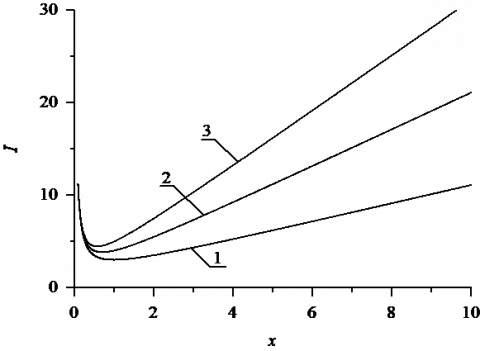Figure 1d. Dependence of the cost function on the quantity of product at different values of maintaining of products d

## 2a.png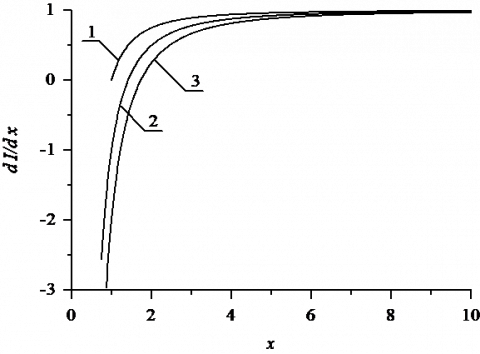Figure 2a. Dependence of derivative of the cost function on the quantity of product at different values of organizational cost a

## 2b.pngFigure 2b. Dependence of derivative of the cost function on the quantity of product at different values of intensity of demand b

## 2c.png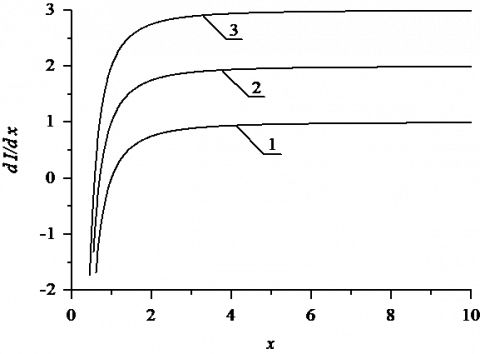Figure 2c. Dependence of the derivative of cost function on the quantity of product at different values of maintaining of products d

## 3a.png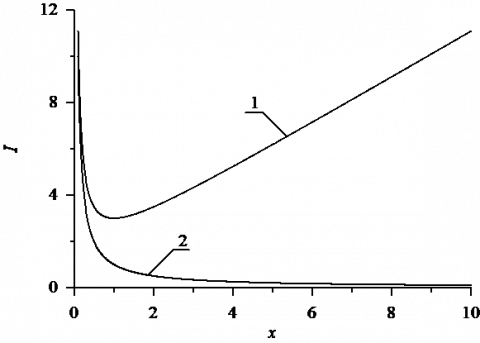Figure 3a. Dependence of the cost function on the quantity of product in comparison with the first term of the function

## 3b.pngFigure 3b. Dependence of the cost function on the quantity of product in comparison with the first term of the function

## 3c.pngFigure 3c. Dependence of the cost function on the quantity of product in comparison with the third term of the function

## 4a.pngFigure 4a. Dependence of derivative of the cost function on the quantity of product in comparison with the first term of the function

## 4b.pngFigure 4b. Dependence of derivative of the cost function on the quantity of product in comparison with the first term of the function

Farther we analyze derivative of cost function on the quantity of product for the same limiting cases. Figures 4a and 4b show the derivative (2) in comparison with the first and the second terms of the function.

3. Conclusion

In this paper, we introduce a model for description of inventory management. Based on this model a condition is formulated to minimize the attendant costs. The condition has been formulated in common form and concrete value of minimal value of attendant costs depend on organizational cost, intensity of demand, cost of the considered products and cost of maintaining of products (see Eq. (2)).

References

 Saleh, A.S., Halili, E., Zeitun, R., Salim, R. (2017). Global financial crisis, ownership structure and firm financial performance: An examination of listed firms in Australia. Studies in Economics and Finance, 34(4): 447-465.

 Orlando, A., Parker, G. (2018). Valuation and risk assessment of a portfolio of variable annuities: A vector autoregression approach. Journal of mathematical finance, 8(5): 349-371

 Dar, A.B., Shah, F.A. (2014). Are eurozone fixed income markets integrated? An analysis based on wavelet multiple correlation and cross correlation. Economics Research International Article ID 219652.

 Nathaphan, S., Chunhachinda, P. (2014). Estimation risk modeling in optimal portfolio selection: An empirical study from emerging markets. Economics Research International Article ID 340181.

 Wu, C., Hung, W.Z. (2015). Economics research international Article ID 678927.

 Mandal, S. (2017). The influence of organizational culture on healthcare supply chain resilience: Moderating role of technology orientation. Journal of Business and Industrial Marketing, 32(8): 1021-1037. https://doi.org/10.1108/JBIM-08-2016-0187

 Bagheri, N., Haghighi, H.K. (2017). A comparison study of ADI and LOD methods on option pricing models. Journal of Mathematical Finance, 7(2): 275-290. https://doi.org/ 10.4236/jmf.2017.72014

 Ple, L., Lecocq, X. (2015). Customers as creative resources: their influence on firm freedom. Journal of Business Strategy, 36(4): 11-22. https://doi.org/10.1108/JBS-05-2014-0051

 Lynette, J.R., Iain, A.D. (2013). Where's the strategic intent in key account relationships? Journal of Business and Industrial Marketing, 28(2): 111-124. https://doi.org/10.1108/08858621311295254

 Frenod, E., Menard, P., Safa, M. (2018). Two optimization problems of a continuous-in-time financial model. Jour-nal of Mathematical Finance, 8(1): 27-42. https://doi.org/10.4236/jmf.2017.72014

 Wu, Ch.W., Hung, W.Zh. (2010). Real national income average growth rate: A novel economic growth and social fair evaluation index. Economics Research International, Vol. 2010, Article ID 678927. http://dx.doi.org/10.1155/2015/678927

 Yaghoubi, R., Yaghoubi, M., Locke, St., Gibb, J. (2015). Mergers and acquisitions. Studies in Economics and Finance, 33(3): 437-4641. https://doi.org/10.1108/SEF-07-2015-0165

 Mehrotra, S.N., Carter, D.R. (2017). Forecasting performance of lumber futures prices. Economics Research Inter-national, Vol. 2017. Article ID 1650363. https://doi.org/10.1155/2017/1650363

 Villeneuve, St., Warin, X. (2014). Optimal liquidity management and hedging in the presence of a non-predictable investment opportunity. Mathematics and Financial Economics, 8(2): 193-227. https://doi.org/10.1007/s11579-013-0108-7

 Mertzanis, Ch. (2017). Short selling regulation, return volatility and market volatility in the Athens Exchange. Studies in Economics and Finance, 34(1): 82-104. https://doi.org/10.1108/SEF-06-2015-0157

 Korn, G., Korn, T. (1968). Mathematical handbook for scientists and engineers. Definitions, theorems and formulas for reference and review. McGraw-Hill Book Company, New York, Second edition.